## Monday, November 24, 2014

### Data Mining in Excel Part 30: Cross-Validation

Today, we're going to talk about the final tool in the "Accuracy and Validation" segment of the Data Mining Ribbon, Cross-Validation.Cross-Validation
Imagine you have a data set with 10,000 rows.  Then, you randomly assign 1,000 rows to each of 10 buckets.  Next, you create a model using 9 of the buckets (9000 rows) as training data and 1 bucket (1000 rows) as testing data.  Then, you repeat this process for the using each of the remaining 9 buckets as testing data.  After this is done, you have 10 models using parts of the same data set, which means you have 10 different predictions for each row.  Then, you can compare the predictions and see how much they deviate from each other.  If they are all very close to each other, then the model is "good".  If some of them are very far apart, then the model may not be appropriate for the data you have.  You can also compute a single prediction by combining all or part of your 10 predictions into a single value.  This process is called Cross-Validation.  For more information, read this.  Let's see it in action.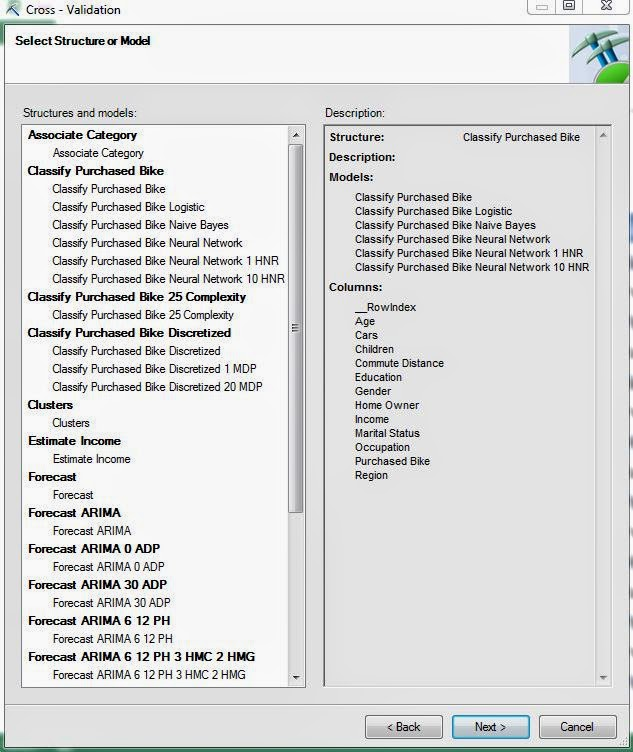Select Structure
First, we need to select the structure or model we would like to use.  Unlike the previous methods, this technique can technically use Association Rules Models as well.  However, the structure must have a variable with the "Predict" flag, which doesn't come from the Association Rules Model.  Therefore, we're not sure how you could use this technique on an Association Rules Model.  Let's move on.Select Parameters
Next, we need to select our parameters to be used.  Fold Count refers to the number of partitions we create using the data.  In our earlier example, we used 10.  You also need to define how many rows you would like to be used for consideration.  The larger the number of rows, the longer the algorithm will take.  Then you need to define with value you are looking, Purchased Bike = Yes in our case.  Finally, you need to decide what probability defines a "True".  These algorithms don't actually assign a True/False value to the field, they assign a probability.  The higher the probability, the more likely the customer will buy a bike.  For now, let's just set this value at .5, like the flip of a coin.  Finally, let's check out the results.Prediction Summary (.5 TT)
We rearranged the tables to make it easier to read.  Here, we have tables telling us the average number of true positives, false positives, true negatives, and false negatives across the 10 folds, as well as the associated standard deviations.  We want to have high numbers for True scores and low number for False scores.  We also want standard deviations to be small because this implies that the models are robust, i.e. aren't heavily influenced by differences in the data.  Looking at this carefully, we see that the Naive Bayes Model performs well on all fronts and has a pretty low standard deviation.  This, combined with the fact that this model also performed well on the other previous Model Validation tests, leads us to believe that the Naive Bayes Model is a good fit for this type of analysis.  However, this is just our opinion and is definitely up to interpretation.  Let's check out the next set of metrics further down the sheet.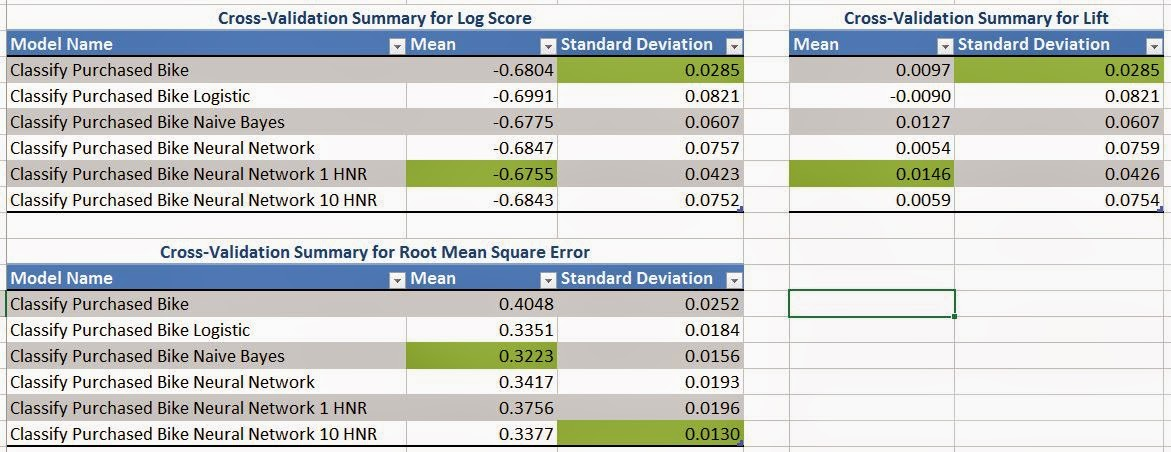Metric Summary (.5 TT)
Here, we have 3 metrics displayed, Log Score, Lift, and Root Mean Squared Error.  We won't go into detail about what these metrics mean.  If you want to know you can read Log Score, Lift, and Root Mean Squared Error.  Basically, we want Log Score and Lift to be high, while keeping Root MSE low.  Of course, we also want the standard deviations to be small.  Personally, we don't feel like these metrics are as important as the prediction values we saw previously.  So, we'll just use them to confirm that the Naive Bayes Model is reasonable, and they do.  Before we finish, let's check the Prediction Summary for different values of Target Threshold to ensure stability of our Naive Bayes Model.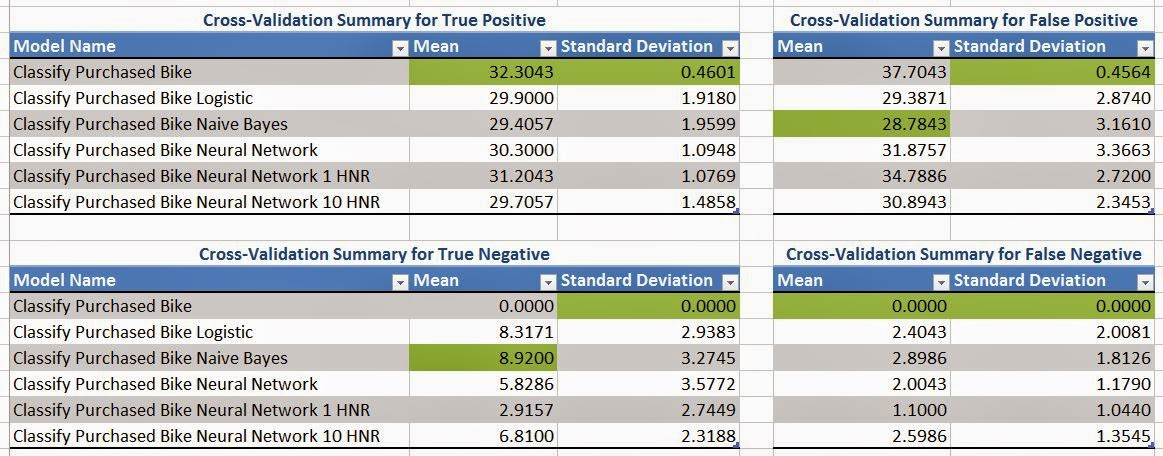Prediction Summary (.2 TT)Prediction Summary (.8 TT)
By looking at these charts, we see that the Naive Bayes Model seems reasonable in all cases.  This leads us to conclude that the Naive Bayes Model is the model we will use to predict which customers will buy our bikes.  Stay tuned next week where we'll be talking about our final topic, Querying your Models.  Thanks for reading.  We hope you found this informative.

Director, Consumer Sciences
Consumer Orbit
llewellyn.wb@gmail.com

## Monday, November 17, 2014

### Data Mining in Excel Part 29: Profit Chart

Today, we're going to talk about the next in the Microsoft suite of Model Validation tools, Profit Chart.Profit Chart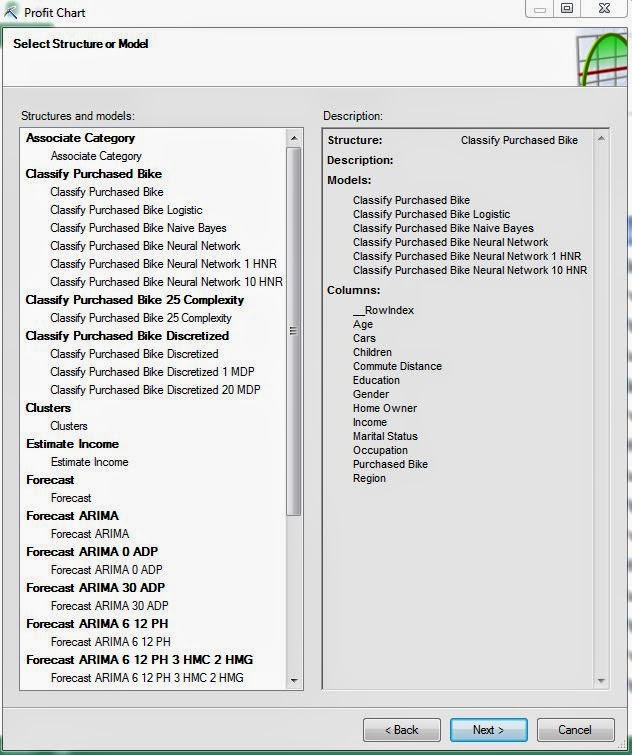Select Structure
First, we need to select our structure or model.  Just like the previous two posts on Accuracy Charts and Classification Matrices, this chart cannot be used on models that were built using the Association Rules or Time Series algorithms.  Let's move on.Select Parameters
Here, we can input the cost of our campaign.  First, we need to decide which value we're looking for.  In our case, we want to find people who will buy a bike.  Next, you input the number of potential customers, 50000 in our case.  Then, you input the fixed cost of creating the campaign, \$5000 for us.  Now, you input the individual cost to advertise to a person, \$10 in this example.  Lastly, you need to define how much money you would make it you sold a bike, \$15 per bike.  This is a great start.  However, in the real world, we get discounts for dealing in bulk.  So, what if an advertiser will give you a discount to create a certain amount of advertisements.  If we click the "..." beside the "Individual Cost" box, we can input that.Individual Cost (Advanced)
Here, we're saying that the first 1000 flyers will cost \$10 per flyer, the next 9000 will cost \$7.50 per flyer and everything above that costs \$5 per flyer.  Let's keep going.Select Data Source
Finally, we need to choose our data source.  As usual, we strongly advise against testing the model using the same data that you used to train the model.  Let's check out the results.Profit Chart (Purchased Bike)
This chart requires a little background.  Imagine that you used these algorithms to assign a probability to each prospective customer (which we haven't learned how to do yet).  This probability tells you how likely that customer is to buy a bike from you.  Then, you order these customers according to those probabilities, highest probability to lower probability.  This ordering is how the horizontal (X) axis of this chart works.  Let's pick 30%.  This point says that if you advertised to the top 30% of your prospective customers as defined by this model, you would make Y dollars.  So, the goal is to see what percentage of our population we need to advertise to in order to maximize our profit.  We see that the blue line (random) is basically the worst performer out of the bunch, followed closely by the red line (Decision Tree Model).  The rest of the models seem to be pretty tightly packed.  The algorithm gives us an easier chart to read.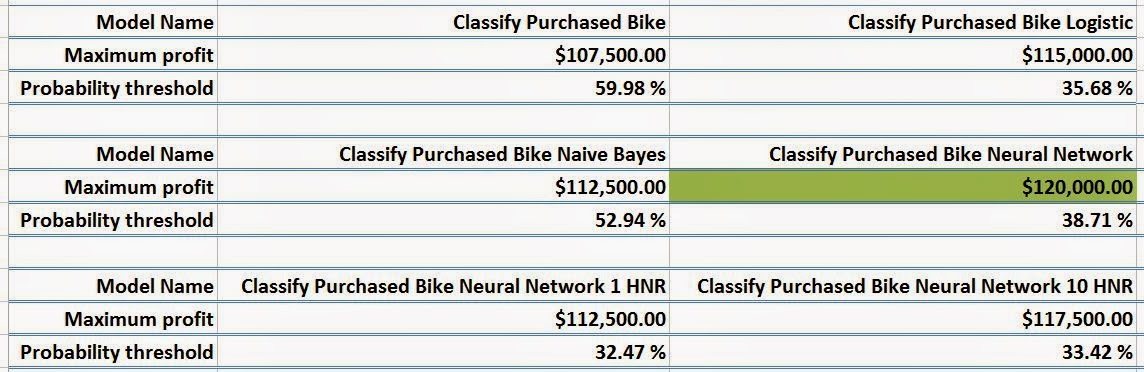Maximum Profit per Model
This table was originally on a single line in the Excel worksheet.  We moved it around for easy readability.  We see that the best model is the Neural Network (again).  Followed closely by each of the other models.  This table also tells us what percentage of the population we would have to advertise to in order to make that amount of money.  Compare the Neural Network Model (Middle Right) to the Neural Network 10 HNR Model (Bottom Right).  If we advertise to 5% more people, we make \$2500 more.  This isn't an incredibly large amount.  If there's some subtle reason why we wouldn't want to advertise to that many people, then perhaps we should pick the Neural Network 10 HNR Model despite the fact that it's not worth as much.

Now that we've gone through three different Model Validation procedures, we should have a good grasp on how to analyze these models to see which perform the best.  Stay tuned for our next post where we'll be talking about Cross-Validation.  Thanks for reading.  We hope you found this informative.

Director, Consumer Sciences
Consumer Orbit
llewellyn.wb@gmail.com

## Monday, November 10, 2014

### Data Mining in Excel Part 28: Classification Matrix

Today, we're continuing our discussion about Model Validation by looking at the Classification Matrix.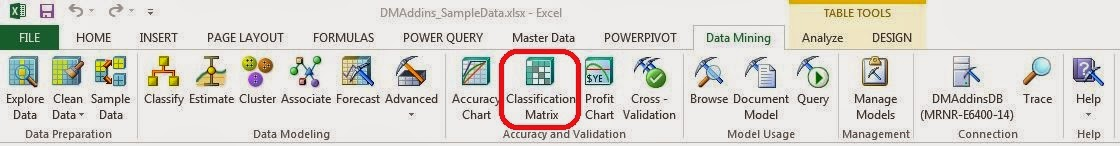Classification Matrix
A Classification Matrix is very useful for determine how well a model predicts values by comparing the predictions to the known values.  Simply put, there are four outcomes for a binary (Yes/No) variable.  First, the actual value is No and the prediction is No (this would be a correct prediction).  Second, the actual value is Yes and the prediction is Yes (this would also be a correct prediction).  Third, the actual value is No and the prediction is Yes (this would be an incorrect prediction and a Type I Error).  Lastly, the actual value is Yes and the prediction is No (this would be an incorrect prediction and a Type II Error).  For more information on Type I and Type II Errors, read this.  A good model reduces the chance of error and increases the chance of correct predictions.  We can display this in a simple grid format.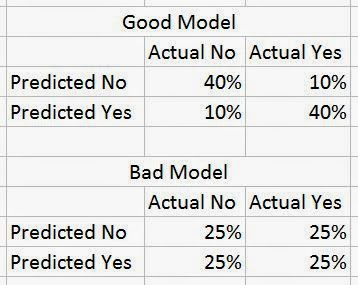Classification Matrices (Mock-up)
The first model has a high chance (80%) of correct predictions (No/No and Yes/Yes) and a low chance (20%) of incorrect predictions (No/Yes and Yes/No), while the bad model only has a 50/50 chance of predicting the correct value.  There's not really a golden rule for how well a model needs to be able to predict values.  For instance, it's not fair to say that a model needs at least a 75% chance of predicting correctly in order to called "Good".  How much is good enough depends on the data and the problem.  For instance, in some marketing campaigns, you have success rates at low as 5% or 10%.  The only thing needed for a good model is that it gleams reasonable insight from actual data.  Everything else comes from good data stewardship and model validation.  Let's see it in action.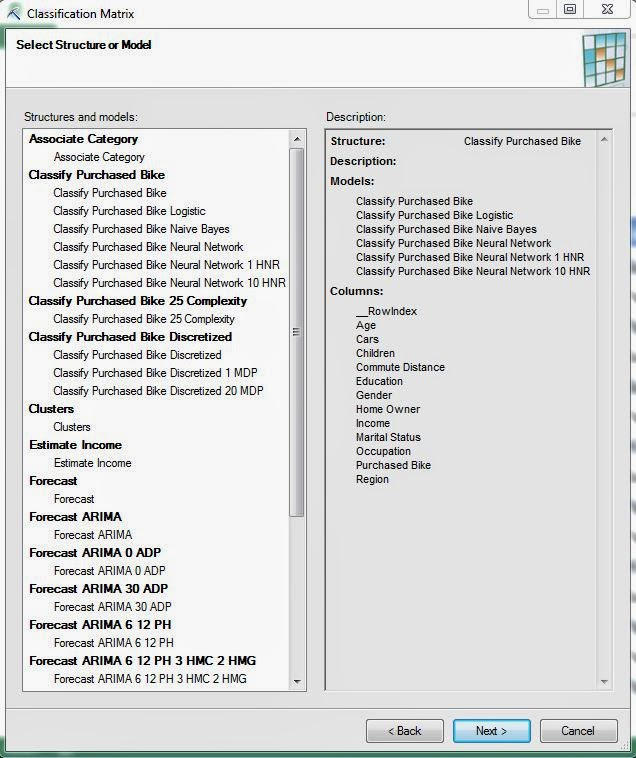Select Structure
The first thing we need to do is pick a model or structure that we would like to create some classification matrices for.  A classification matrix is perfectly valid for a single model, but they are definitely better if you can compare multiple models.  Just like the previous post on Accuracy Charts, this technique will not work on models built using the Association Rules or Time Series algorithms.  Let's keep going.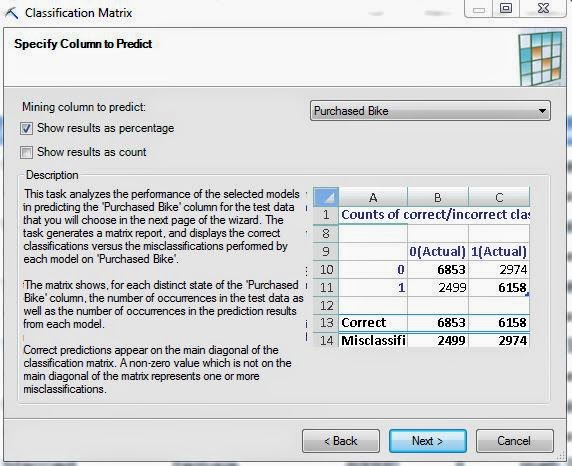Select Column
The next step is to select which column we would like to predict.  Since this structure only has one predictable column, we don't have much choice.  However, we do have the option of seeing percentages, counts, or both.  Personally, we like to see percentages, but the choice is yours.  Let's move on.Select Data Source
Again, we have the option of choosing a data source.  We defined test data for exactly this reason; so, there's no harm in using it.  Just remember, it's not acceptable to use the same data that you used to train the model.  Let's check out the results.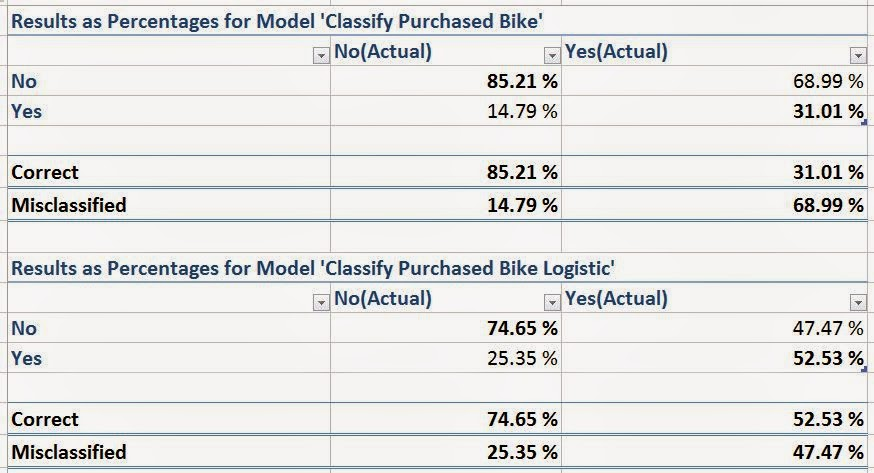Classification Matrices (Purchased Bike)
We have a classification matrix for each model in the structure.  The percentages in these matrices are calculated slightly differently than in our mock-up.  In these matrices, each column adds up to 100%.  This is because we can conceptualize each Actual value as a unique outcome.  After all, a single person can't be a bike buyer while also not being a bike buyer.  We prefer this method, but there are quite a few others as well.  You're free to google them if you want.  Just on the first page of results we saw at least four distinct types of classification matrices.

An important thing to note from these models is how well they predict the outcome you are looking for.  In our case, we want to find bike buyers.  We don't care about the non-bike buyers.  So, all we need to focus on is the Yes column.  In that case, the Logistic Model (the bottom one) seems to be a pretty poor model.  It's barely better than flipping a coin!  However, if you compare it to the Decision Tree Model (the top one), you'll see that 52% is not a bad percentage.  Let's take a look at the last type of chart.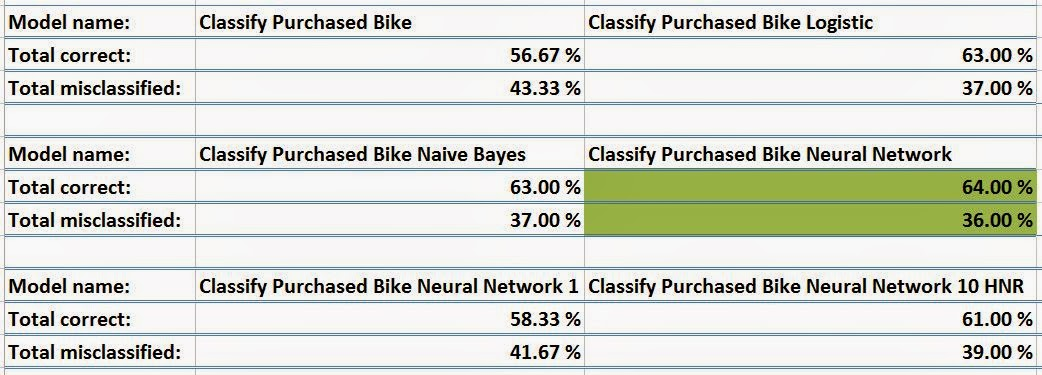Multi-Model Classification Matrix
This matrix was originally on a single line in Excel.  However, it was difficult to screenshot.  So, we used some Excel magic to put it on multiple lines.  This matrix shows us how well each model compares, regardless of which outcome we care about.  It also highlights the "best" model in green.  If we look back down at our single model classification matrices, we see that the Naive Bayes Model actually has the highest Yes/Yes classification percentage at 53.8%.

So, the accuracy chart told us to use the Logistic Model, the single-model classification matrix told us to use the Naive Bayes Model and the multi-model classification matrix told us to use the Neural Network Model.  Which model is the right one?  There's no real answer to that question.  It's up to each of us to make that decision on our own.  In the real world, there is rarely a "best" answer.  Simply choose the model that works well for you.  Keep an eye out for our next post where we'll be talking about Profit Charts.  Thanks for reading.  We hope you found this informative.

Director, Consumer Sciences
Consumer Orbit
llewellyn.wb@gmail.com

## Monday, November 3, 2014

### Data Mining in Excel Part 27: Accuracy Chart

Today, we're going to talk about a number of ways that you can use to compare models against each other using the Accuracy and Validation Segment of the Data Mining Ribbon.  Specifically, we'll be using the Accuracy Chart.Accuracy Chart
The Accuracy Chart tells you how large your sample would need to be to predict a certain number of values.  Let's say that we want to find customers who will buy our bikes.  We have a sample of 100 customers and we know that 50 of them will buy a bike.  How many of these customers do we need to talk to find our 50 bike buyers?  If we had a perfect model, we would only want to talk to the 50 bike buyers.  If we flipped a coin, i.e. random chance, we would theoretically need to talk to all 100 customers to find the 50 bike buyers.  Therefore, we want our model to be somewhere in between.  The model can't be better than perfect.  But, it needs to do better than random chance.  Let's look at this graphically.Accuracy Chart (Mock-up)
With a perfect model, we know that the first 50 customers we talk to will be bike buyers.  However, since only half of the customers are bike buyers, the random chance model will give us a bike buyer half of the time.  In layman's terms, a steeper line is better.  Let's try to make this using our models.Select Structure
First, we want to select the structure that we would like to analyze.  We typically want to choose a structure with multiple models in order to have a real comparison.  However, there's nothing stopping you from using this on a single model.  This will allow you to compare that model again the Perfect Model and Random Chance.  It should be noted that the Accuracy Chart cannot be built using models with the Association Rules or Time Series Algorithms.  Let's move on.Select Column
Now, we need to choose which value we are looking for.  In our case, we want Purchased Bike = Yes.Select Data Source
Next, we need to choose our data source.  Remember when we were building the models and we were asked to set aside a Testing Set?  This is where we can access that data.  If you chose not to set aside a Training Set, you can always specify a new data source as well.  However, it is typically frowned upon to test a model using the same data you used to train the model.  This is designed to prevent "Overfitting".  Basically, "Overfitting" is when your model is good at predicted data it has seen, but isn't very good at predicting new data.  For more information on overfitting, read this.  Let's check out the results.Lift
The first table we see is a set of lift values.  Basically, Lift is a measure of how good the model is compared to Random Chance.  A lift of less than 100% would be worse than random, while a lift above 100% is better.  For more information on lift, read this.  We see that the basic model, which used the Decision Tree algorithm, was actually the worst model our of all of them, while the Logistic Model was the best.  The Decision Tree model has a lift of 110%.  This means that it would be perfectly acceptable to use this model.  However, since we put in the extra effort to create a few more candidate models, we got an improvement of almost 10%!  This just goes to show that you shouldn't stick with your first model just because it was the easiest to build.  Let's check out the chart.Accuracy Chart (Purchased Bike)
Most of the models are pretty tightly clustered.  This means that there's not a huge difference between them.  However, we can definitely see that the green line (Decision Tree) falls off pretty badly when we look at larger percentages.  The key here is to find out how many people you plan on sampling, and picking the model that performs best at that percentage.  If you have a couple of close models, you can pick multiple models and combine the results.  For instance, you can pick the top 3 models, and average the results.  If 2/3 say Yes, then that person is likely to buy a bike.  If 2/3 say No, then don't waste your time on that person.  Further down in the worksheet is a table with all of the numbers in it if you'd like to look at that.

We're finally starting to wrap up our analysis with some Model Validation.  Hopefully, we've piqued your interest to go and use this tool with your data.  Stay tuned for the next post where we'll be talking about the Classification Matrix.  Thanks for reading.  We hope you found this informative.